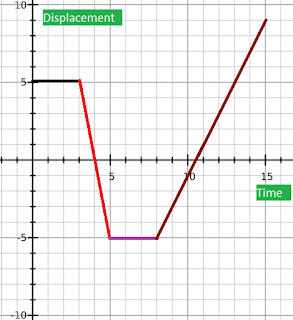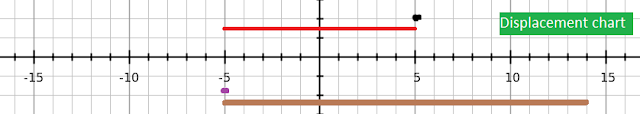## Pages

### 2.1 Kinematics (Graphical methods)

Here we'll discuss something about the graphical representation of the kinematics. In particulars,

1.Graphical methods to represents the displacement, speed, velocity and acceleration.
2.Slope of a displacement-time graph to find the velocity
3.Finding the displacement from area under velocity-time graph.
4.Slope of a velocity-time graph to find the acceleration.

Firstly, Here's video to understand Graphical methods to represents motion.

Slope of a displacement-time graph to find the velocity: One of the easiest way to record the observation is to do it graphically. Let us say that from our recorded datas we have the folloing plot of the graphs as shown below. What does this imply?Displacement vs Time Plot

The given above graph represent the numerical values in a graphical form. We now try to extract information from the graph. It is important to remember the definition of the relavent kinematics variables to extract the information.
"In the first three seconds an object is at rest(represented by the black line), in the next two seconds the object moves towards the refence zero point and to the opposite direction(represented in the red line), again for the next three seconds the objects is at rest(represented by the purple line) and finally the object again moves towards the zero point and goes to the positive direction for seven seconds."

Displacement chart would look something like this, (color code is followed with respect to above graph.)Another important things we need to be able to do is to find the velocity from the displacement-time graph. The slope of the displacement-time graph is the velocity of the objects.

Ex. find the velocity from the given above displacement-time graph.(i'll leave calculation to you.)
Soln:for black line=0m/s,   for red line =5m/s,    for purple line=0m/s,   for brown line= 2.333 m/s

Finding the displacement from area under velocity-time graph: If we are given a velocity-time then we can find the displacement covered by finding the area under the graph. For example you can refer to the following graph.

EX. Find the total displacement covered in 15 sec?
soln: 87.5m (hint: find the area under the orange line and the pink line and add it)

Slope of a velocity-time graph to find the acceleration: If we want to find the acceleration of the object from the graph(velocity-time) above, we simply find the slope of the functions. In the case of the orange line on the graph, the acceleration(slope) is (1$m/s^{2}$) and for the pink line the acceleration(slope) is(0.5$m/s^{2}$). It is clear that in this case the acceleration is constant from (0-5)sec and from (5-15)sec.